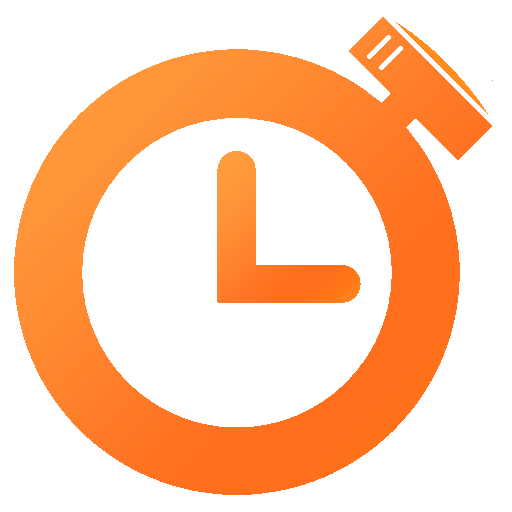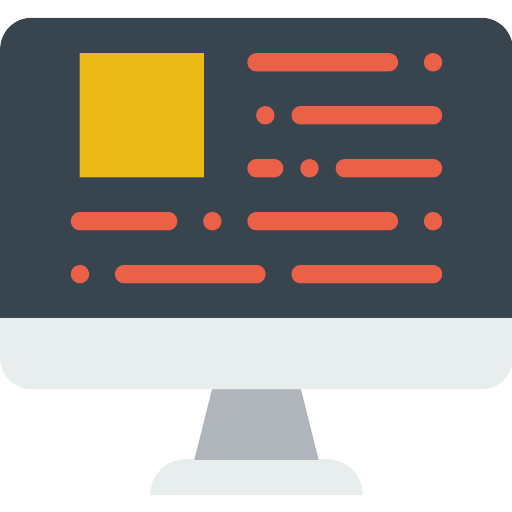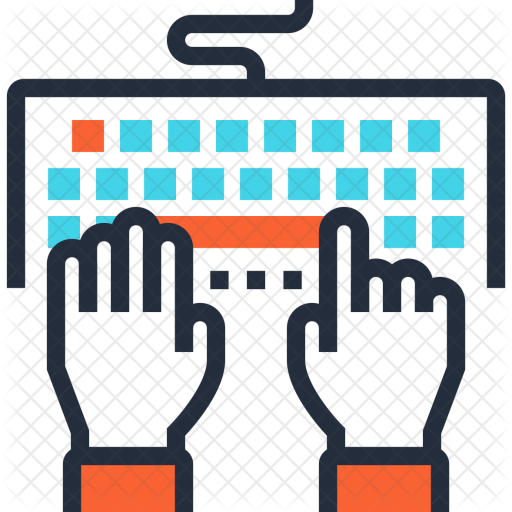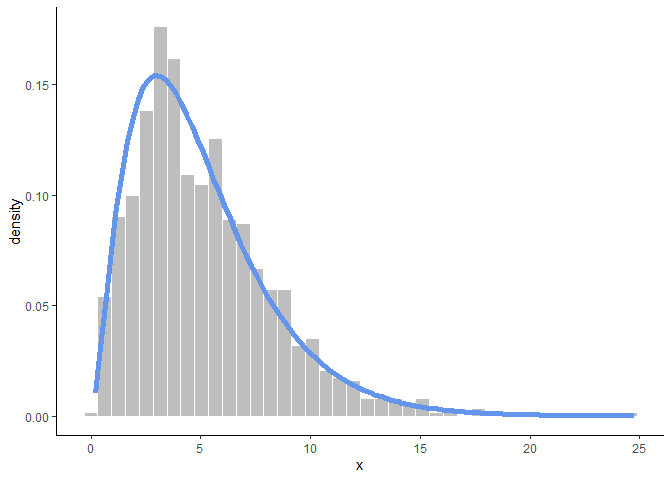# Distributions and SimulationTime Estimates:Videos: 30-60 minReadings: 10 minActivities: 45 minCheck-ins: 3

## Review: Statistical DistributionsRequired Video: Review of Statistical DistributionsOptional Tutorial: Interactive Random Variable Creator

## Simulating in RRequired Video: Simulating Data in ROptional Reading: RProg Ch 20: SimulationCheck-In 1: The r, p, q, and d functions

Try to predict what the following outputs will be WITHOUT running the code in R. Drawing pictures of the relevant distributions may help.

(Yes, it is very easy to “cheat” on this question. But this is for your practice, and I recommend you give it some thought.)

``````## a
punif(0.674)

## b

pnorm(2)
qnorm(.975)

## c

pchisq(0, df = 12)

## d

qt(10, df = 16)

## e

dbinom(2, size = 2, prob = .4)
pbinom(1, size = 2, prob = .6)``````

## Preparing for the Lab

### The Central Limit Theorem

If you do not recall the Central Limit Theorem from you previous classes - or if you would benefit from a refresher:Optional Video: The CLTCheck-In 2: The CLT

Explain the idea behind the CLT in your own words, using as little jargon/vocabulary as you can.

### Plotting distributions

Here is the code that made one of the plots from the lecture video:

``````my_samples <- data.frame(x = rchisq(1000, df = 5))

ggplot(my_samples, aes(x)) +
geom_histogram(bins = 40, aes(y = ..density..), fill = "grey", col = "white") +
stat_function(fun= ~dchisq(.x, df = 5), col = "cornflowerblue", lwd = 2) +
theme_classic()``````